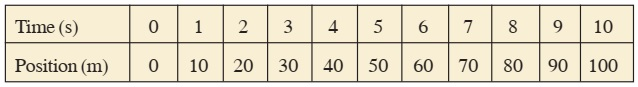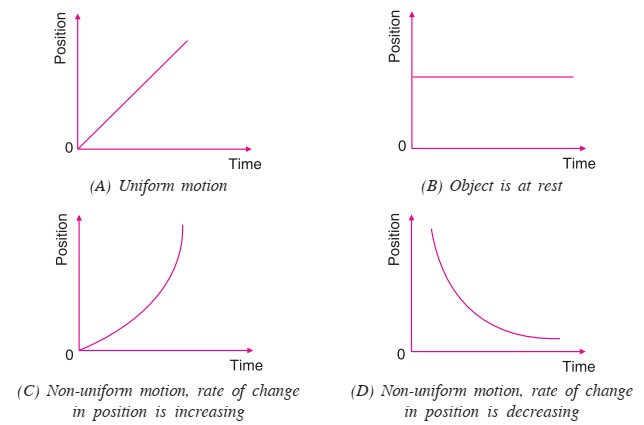# Position-Time Graph

It is easy to analyze and understand motion of an object if it is represented graphically. To draw graph of the motion of an object, its position at different times are shown on y-axis and time on x-axis.

Example: Positions of an object at different times are given in table.In order to plot position-time graph for data, represent time on horizontal axis and position on vertical axis drawn on a graph paper. Next, choose a suitable scale for this. For example, one division on horizontal axis represents 1 s of time interval and one division on vertical axis represents in 10 m, respectively. If you join different points representing corresponding position time data, you get straight line. This line represents the position-time graph of the motion.Displacement of the object in 1st second, 2nd second, and so on is 10 m. In 10 second, the displacement is 100 m. Therefore, velocity is 100 m / 10 s = 10 ms–1 for the whole course of motion.

Velocity during 1st second = 10 ms–1 and so on.

Thus, velocity is constant i.e., equal to 10 ms–1 throughout the motion. The motion of an object in which velocity is constant, is called uniform motion.

Like position-time graph, you can also plot displacement-time graph. Displacement is represented on the vertical axis and time interval on the horizontal axis.